# 基于统计建模下共享单车的调查分析与前景预测 Investigation and Prospect Forecast of Bicycle Sharing Based on Statistical Modeling

• 全文下载: PDF(970KB)    PP.39-47   DOI: 10.12677/SA.2019.81006
• 下载量: 210  浏览量: 502   科研立项经费支持

As we enter the new era, bicycle sharing has become synonymous with green travel and easy health. However, any new type of business activity always comes with chaos in the beginning such as barbarous growth and disordered management. Bicycle sharing is no exception. Situations such as disordered placement and severe damage of bicycles are troubling the users. There are many factors affecting the satisfaction of users. This paper studies the basic characteristics of users and the objective factors that affect user satisfaction. Firstly, the independent test and the lining analysis are applied to the basic characteristics of the user, and the exploratory factor analysis and the confirmatory factor analysis are applied to the influencing factors. Secondly, the Logistic model is used to predict from the two aspects of the user’s basic characteristics and influencing factors. The results show that there is a significant relationship between the basic characteristics of users and user satisfaction. Safety hazards and green environmental protection have significant effects on user satisfaction.

1. 引言

2. 测量工具与模型

2.1. 李克特量表

2.2. 结构方程模型

3. 数据分析与处理

3.1. 问卷组成

1) 使用者的基本特征：性别、年龄、职业等；

2) 使用者的用车特征：使用频率、用车用途、用车期望等；

3) 客观影响因素：由23个题项组成的研究变量。

3.2. 数据清洗与检验

4. 模型建立与实证分析

4.1. 使用者认同度的列联分析

4.2. 探索性因子分析Table 2. Basic feature type of usersTable 3. Basic feature test valueTable 4. Principal component cumulative contribution rate

Continued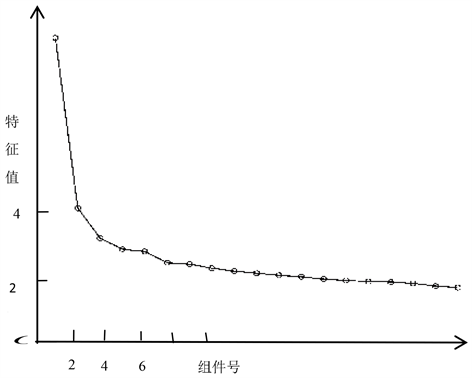Figure 1. Gravel map

$\begin{array}{l}{F}_{1}=-0.049{x}_{1}-0.086{x}_{2}+0.297{x}_{3}+0.296{x}_{4}+0.275{x}_{5}+0.272{x}_{6}+0.020{x}_{7}\\ \text{\hspace{0.17em}}\text{\hspace{0.17em}}\text{\hspace{0.17em}}\text{\hspace{0.17em}}\text{\hspace{0.17em}}\text{\hspace{0.17em}}\text{\hspace{0.17em}}-0.121{x}_{8}-0.077{x}_{9}-0.013{x}_{10}+0.063{x}_{11}+0.095{x}_{12}+0.130{x}_{13}-0.018{x}_{14}\\ \text{\hspace{0.17em}}\text{\hspace{0.17em}}\text{\hspace{0.17em}}\text{\hspace{0.17em}}\text{\hspace{0.17em}}\text{\hspace{0.17em}}\text{\hspace{0.17em}}+0.045{x}_{15}+0.034{x}_{16}+0.030{x}_{17}-0.206{x}_{18}-0.233{x}_{19}\end{array}$ ,

$\begin{array}{l}{F}_{2}=-0.065{x}_{1}-0.050{x}_{2}+0.017{x}_{3}-0.108{x}_{4}-0.006{x}_{5}+0.046{x}_{6}-0.199{x}_{7}\\ \text{\hspace{0.17em}}\text{\hspace{0.17em}}\text{\hspace{0.17em}}\text{\hspace{0.17em}}\text{\hspace{0.17em}}\text{\hspace{0.17em}}\text{\hspace{0.17em}}-0.001{x}_{8}-0.137{x}_{9}+0.125{x}_{10}+0.063{x}_{11}+0.322{x}_{12}+0.289{x}_{13}+0.266{x}_{14}\\ \text{\hspace{0.17em}}\text{\hspace{0.17em}}\text{\hspace{0.17em}}\text{\hspace{0.17em}}\text{\hspace{0.17em}}\text{\hspace{0.17em}}\text{\hspace{0.17em}}-0.123{x}_{15}-0.096{x}_{16}-0.095{x}_{17}+0.209{x}_{18}+0.230{x}_{19}\end{array}$ ,

$\begin{array}{l}{F}_{3}=-0.047{x}_{1}-0.077{x}_{2}-0.085{x}_{3}-0.021{x}_{4}-0.063{x}_{5}+0.272{x}_{6}-0.112{x}_{7}\\ \text{\hspace{0.17em}}\text{\hspace{0.17em}}\text{\hspace{0.17em}}\text{\hspace{0.17em}}\text{\hspace{0.17em}}\text{\hspace{0.17em}}\text{\hspace{0.17em}}-0.290{x}_{8}+0.012{x}_{9}-0.178{x}_{10}-0.155{x}_{11}-0.163{x}_{12}-0.140{x}_{13}-0.040{x}_{14}\\ \text{\hspace{0.17em}}\text{\hspace{0.17em}}\text{\hspace{0.17em}}\text{\hspace{0.17em}}\text{\hspace{0.17em}}\text{\hspace{0.17em}}\text{\hspace{0.17em}}+0.340{x}_{15}+0.325{x}_{16}+0.323{x}_{17}+0.132{x}_{18}+0.167{x}_{19}\end{array}$ ,

$\begin{array}{l}{F}_{4}=0.007{x}_{1}-0.003{x}_{2}-0.077{x}_{3}+0.013{x}_{4}+0.016{x}_{5}+0.026{x}_{6}+0.436{x}_{7}\\ \text{\hspace{0.17em}}\text{\hspace{0.17em}}\text{\hspace{0.17em}}\text{\hspace{0.17em}}\text{\hspace{0.17em}}\text{\hspace{0.17em}}\text{\hspace{0.17em}}+0.077{x}_{8}+0.430{x}_{9}+0.306{x}_{10}+0.193{x}_{11}-0.062{x}_{12}-0.070{x}_{13}-0.093{x}_{14}\\ \text{\hspace{0.17em}}\text{\hspace{0.17em}}\text{\hspace{0.17em}}\text{\hspace{0.17em}}\text{\hspace{0.17em}}\text{\hspace{0.17em}}\text{\hspace{0.17em}}-0.009{x}_{15}-0.015{x}_{16}-0.027{x}_{17}-0.092{x}_{18}-0.108{x}_{19}\end{array}$ ,.Table 6. Multiple linear regression parameter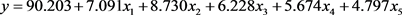(2)

4.3. 路径分析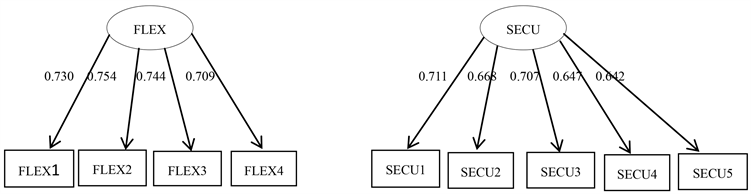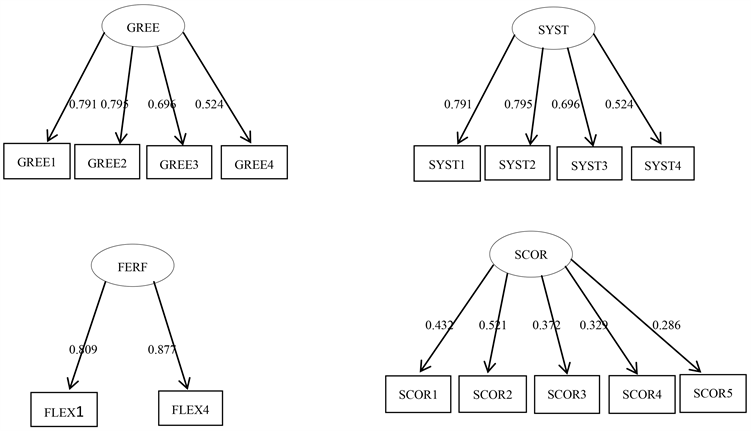Figure 2. Measurement modeling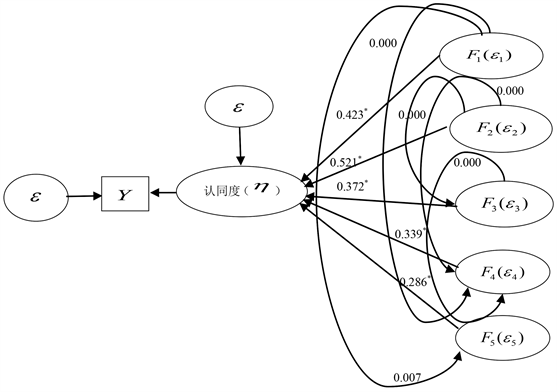注：**表示模型在0.01水平下显著。

Figure 3. Structural Equation Modeling

4.4. Logit模型构建——前景预测

$Logit{P}_{5}=\frac{\mathrm{exp}\left(3.330+1.829{F}_{1}+1.856{F}_{2}+2.313{F}_{3}+1.933{F}_{4}\right)}{1+\mathrm{exp}\left(3.330+1.829{F}_{1}+1.856{F}_{2}+2.313{F}_{3}+1.933{F}_{4}\right)}$ (3)

$\mathrm{ln}\left(\frac{P\left(y=5|x\right)}{P\left(y=1|x\right)}\right)=3.330+1.829{F}_{1}+1.856{F}_{2}+2.313{F}_{3}+1.933{F}_{4}$ (4)

$Logit{P}_{4}=\frac{\mathrm{exp}\left(4.265+0.758{F}_{1}+1.619{F}_{2}+2.313{F}_{3}+1.933{F}_{4}\right)}{1+\mathrm{exp}\left(4.265+0.758{F}_{1}+1.619{F}_{2}+2.313{F}_{3}+1.933{F}_{4}\right)}$ (5)

$\mathrm{ln}\left(\frac{P\left(y=4|x\right)}{P\left(y=1|x\right)}\right)=4.265+0.758{F}_{1}+1.619{F}_{2}+2.313{F}_{3}+1.933{F}_{4}$ (6)

4.5. 结论与建议

  共享单车累计投放超1000万辆管理新政将陆续出台——中新网[EB/OL]. https://www.chinanews.com/cj/2017/05-24/8232308.shtml, 2017-05-24.  吴明隆. 结构方程模型: AMOS的操作与应用[M]. 重庆: 重庆大学出版社, 2009.  蒋丽芹, 吴宝泰. 基于因子分析法的城市公共自行车系统公众满意度研究——以江苏无锡为例[J]. 哈尔滨商业大学学报: 社会科学版, 2014(4): 73-80.  崔梦蕾. 基于顾客满意度的城市居民对公共自行车出行分析——以武汉市为例[J]. 现代商贸工业, 2011(9): 125-126.